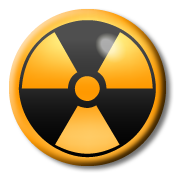Page 1

 1. An atom of 14 C contains protons and neutrons. How many? 6
 number of neutrons number of protons A. 14 6 B. 8 6 C. 6 8 D. 6 14

Look at this diagram of an atom of a common element.(not to scale)

2. What is the atomic number?

A). 3
B). 4
C). 7
D). 10

3. What is thenucleon (mass) number?

A). 3
B). 4
C). 7
D). 10

4. Two isotopes of the same element have

A). the same number of protons but different numbers of neutrons.
B). the same number of protons but different numbers of electrons.
C). the same number of neutrons but different numbers of protons.
D). the same number of electrons but different numbers of protons.

5. An atom that has been ionised has

A). Lost or gained protons
B). Lost or gained electrons
C). Lost or gained a nucleon
D). Lost or gained a neutron

6. A radioactive isotope is a substance that

A). will eventually gain electrons through bonding.
B). will become stable by cooling down.
C). is unstable and will ‘decay’ by losing particles.